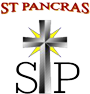Skip to content ↓# Milestones

Bold and Italic Milestones have been taught.

 NUMBER, PLACE VALUE & ROUNDING Y4.114 I can count in multiples of 6, 7, 9, 25 and 1000. Y4.115 I can count backwards through zero to include negative numbers. Y4.116 I know the value of each digit in a 4 digit number. Y4.117 I can order and compare numbers beyond 1000. Y4.118 I can round any number to the nearest 10, 100 or 1000. Y4.119 I can read Roman numerals to 100 (I to C). ADDITION AND SUBTRACTION Y4.120 I can add numbers with up to 4 digits using efficient written methods. Y4.121 I can subtract numbers with up to 4 digits using efficient written methods. Y4.122 I can estimate to check answers to calculations. Y4.123 I can solve two-step addition problems. Y4.124 I can solve two-step subtraction problems. MULTIPLICATION AND DIVISION Y4.125 I can instantly recall X and ÷ facts for multiplication tables up to 12 x 12. Y4.126 I can multiply mentally. Y4.127 I can use place value, known and derived facts to divide mentally. Y4.128 I can multiply three- digit numbers by a one-digit number. Y4.129 I can solve problems involving multiplying and dividing. FRACTIONS Y4.130 I can find equivalent fractions. Y4.131 I can add and subtract fractions with the same denominator. Y4.132 I can write decimal equivalents of any number featuring featuring10ths and 100ths. Y4.133 I can find decimal equivalents to ¼, 1/2 , ¾. Y4.134 I can X and ÷ a number by 10 and 100. Y4.135 I can round decimals with 1 decimal place to the nearest whole number. Y4.136 I can solve simple measure and money problems involving fractions and decimals. MEASURES Y4.137 I can convert between different units of measure (e.g. kilometer to metre; hour to minute). Y4.138 I can measure and calculate the perimeter of a rectilinear figure in centimeters and metres. Y4.139 I can find the area of rectilinear shapes by counting. Y4.140 I can estimate and calculate different measures, including money in pounds and pence. Y4.141 I can read, write and convert time using analogue and digital 12 and 24- hour clocks. GEOMETRY Y4.142 I can classify geometric shapes, including quadrilaterals and triangles, based on their properties and sizes. Y4.143 I know acute, obtuse and reflex angles. Y4.144 I can compare and order angles up to two right angles by size. Y4.145 I can complete a simple symmetrical figure with respect to a specific line of symmetry. Y4.146 I can describe position on a 2-D grid as co-ordinates in the first quadrant. Y4.147 I can translate shapes. Y4.148 I can plot specified points and draw sides to complete a given polygon. DATA Y4.149 I can interpret and present data using line graphs. Y4.150 I can solve ‘comparison’ problems using information presented in bar charts, pictograms, tables and line graphs. Y4.151 I can solve ‘sum’ problems using information presented in bar charts, pictograms, tables and line graphs. Y4.152 I can solve ‘difference’ problems using information presented in bar charts, pictograms, tables and line graphs.